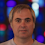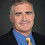## Thursday, December 24, 2020

### Slowest Sorting Algorithms

Radu Gigore tweeted "People are obsessed with finding the best algorithms. What about the worst?" So here's a Christmas gift that keeps giving, the slowest of sorting algorithms.

Before you read on, try to think of the slowest sorting algorithm. No fair spinning its wheels, sleeping or doing unrelated tasks. It should always make progress towards sorting.

Here are some examples, in particular bogosort that generates all permutations until it finds a sorted one. Takes n! time on average.

But we can do much worse. The following redicusort algorithm I got from Stuart Kurtz back in the 90's.

Generate all permutations and then sort those permutations. The sort of the original permutation will be first on the list.

The running time depends on the sorting algorithm you use after generating the permutations.

If you use bubblesort you get a (n!)2 time algorithm.

If you use bogosort you get a (n!)! bound.

What if you just call redicusort recursively? The algorithm will never end. If you want to guarantee termination you need to bound the depth of the recursion. Choose something like an Ackermann function to get a sorting algorithm that always makes progress but not primitively recursive. In general you can get a sorting algorithm that takes longer than any fixed computable function.

1.Steve Mahaney described this problem to me in '83, while we were at a conference at the University of New Hampshire. Michael Ingrassia and I took up the challenge. Ingrassia proposed the "generate all permutations, and look for the sorted one." I contributed the idea of finding the sorted permutation by sorting the list of permutations in lexicographic order. The recursive approach was obvious, as was the problem with it -- that the "sub-problems" were larger than the problem you're trying to solve. We dealt with this by simply limiting the number of recursive steps to the length of the original list, then falling back on quickSort ;-).

This version has the property that sorting an n element list ultimately results in a call to quickSort with the n-fold iterate of factorial applied to n. Thus, sorting a 2 element list applies quickSort to a 2!! = 2! = 2 element list, whereas sorting a 3 element list applies quickSort to a 3!!! = 6!! = 720! element list.

Of couse, you can make this worse still by limited the recursion depth to f n for a suitably defined f, e.g., a total computable function that is total but grows faster than any provably total computable function. We called the result Ingrassia-Kurtz sort, but it never caught on.

2.That's some impressively slow algorithms!

One thing I was wondering is whether one can give a formal distinction between artificially slow and genuinely slow. My first instinct was that the concepts "artificial"/"genuine" are too vague to be made formal. But in that twitter thread I made some proposal that possibly makes sense. It is for circuits rather than algorithms.

In the meantime, I think I have a simpler reformulation: each gate output must be used, each gate must decrease entropy, and each gate must depend on all its inputs. The size of the circuit is the number of gates.

I'll illustrate what I mean by "decrease entropy" on an example. We want to compute the majority function on three variables, called 0,1,2. This circuit does the job:

3 := 0 and 1
4 := 1 and 2
5 := 2 and 0
6 := 3 or 4
7 := 6 or 3

Let's check that the gate (6 := 3 or 4) decreases entropy. Variable 6 is set for 3 inputs (011, 110, 111), so its entropy is 3/8 log(8/3) + 5/8 log(8/5) = 0.954. Variables 3 and 4 are 00 for 5 inputs (000,001,010,100,101), 01 for 1 input (011), 10 for 1 input (110), and 11 for 1 input (111). So, their entropy is 5/8 log(8/5) + 3 (1/8) log(8) = 1.549. Since 1.549>0.954, the gate decreased entropy.

The constraints rule out gates with one input: IDENTITY and NOT do not decrease entropy; ZERO and ONE do not depend on their input. Gates with >2 inputs are not ruled out explicitly, but they are probably not desirable since you want many gates.

I wonder if this makes sense. If so, does it generalize to algorithms?

1.For circuits, if you take the OR of n-input ANDs, one AND for each x such that f(x) = 1, this will give a large circuit for any balanced function that fits your formulation (modulo the nots on the input).

3.How about designing a really slow algorithm that doesn't sort the array correctly? Is this even better

4.This comment has been removed by the author.

5.You can give a "logic twist" to the problem.

Given an unordered sequence of (input) length n
- for all Turing machines Ti of size less than n
-- for all proofs Pj (in Peano arithmetic) of size less than n
--- check if Pj is a proof that Ti is a valid sorter
----- check that Ti has not been used for inputs less than n (in other words, for each n try to use a different Turing machine)
----- simulate the first unused Ti on the input
----- if no new Ti is found use the last one

What is the running time of this sorting algorithm .... ? :-)

[re-posted due to "less than" symbols interpreted as html characters]

1.This is not that bad at all. The size (description length) of the TM that discards inputs of length less than n and sorts the ones of length at least n, is of order log n, so you only need to go through poly(n) short strings, because these all have short proofs. The only question is which TM comes first in your encoding, but this even might be quite a fast algorithm.

2.@dom my idea was to select each time an unused Turing machine (for which there is a proof that it is a sorter).

... not so natural.

In order to make it more natural you can simply consider the length of the input (n) and start the search from the n-th Turing machine and going backward until you find a Turing machine for which there is a valid proof (of size less than n) that it is a sorter, and simulate it on the original input. In this case the "inefficient" backward loop leads to a simulation of all sorters on an infinite number of inputs.

The running time is not bounded by any computable function.

6.I think this question would be more interesting for non-redundant simple comparison trees. By this I mean that in every step we can compare two elements whose relation we do not yet know. How bad can we do for the best input, i.e., how long is the shortest branch? Since every input ends up in a different leaf, sometimes we are done with less than nlog n comparisons.

7.I think I have an answer to this.
If you keep comaring things that you don't know how they compare then the algorihtm has to stop in (n choose 2) steps.

So is there an algorithm the worst case is (n choose 2)
Yes- bubblesort

https://www.sparknotes.com/cs/sorting/bubble/section1/

For average case there may be a diff answer.

8.Here is an exponentially slow Prolog program that reverses a list.
rev([],[]).
rev([A],[A]).
rev([A|XB],[B|YA]) :-
rev(XB,[B|Y]),
rev(Y,X),
rev([A|X],YA).

9.Foundational paper for the field:
Pessimal Algorithms and Simplexity Analysis
Broder and Stolfi, ACM SIGACT 16(3), 1984